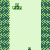# Finding a polygon that contains a point

708
4
03-30-2021 01:02 PMNew Contributor

I'm hoping to automate a process, and doing so involves a python script that can look at points on one feature layer, and determine which polygon, from another feature layer, that point is contained within.

Below, I have included some code that demonstrates my approach. In the end, I would hope for a result of 'True,' if the point was within the polygon, which in this case, it was. However, I get an empty result. This example only tests one point and one polygon, and both were selected because the point is within the polygon.

I've seen many other threads on this subject, but can't seem to find an example that shows how to prepare the point and polygon objects for the use of the 'within()' method.

``````from arcgis.gis import GIS
from arcgis.geometry import Geometry
from arcgis.geometry.filters import within

fl1 = gis.content.get('ece8ed133a2149f98f46fac2042db2f8')
query1 = fl1.layers.query()
resp1 = query1.features
resp1.geometry

# Output
# {'rings': [[[-10397275.0847498, 5628849.44085896],
# [-10385962.4045636, 5628773.00383067],
# [-10386038.8415919, 5618606.87906876],
# [-10397427.9588064, 5618606.87906876],
# [-10397275.0847498, 5628849.44085896]]]}

poly_geometry = Geometry(resp1.geometry)

fl2 = gis.content.get('d14af86121b640f68e050d20f3f0e876')
query2 = fl2.layers.query()
resp2 = query2.features
resp2.geometry

# Output:
# {'x': -10392706.32194956, 'y': 5622351.110157302}

point_geometry = Geometry(resp2.geometry)

print(point_geometry.within(poly_geometry))

# Output:
# None``````

Tags (3)
4 RepliesbyMVP Frequent Contributor

I think you want 'contains' rather than 'within'.byMVP Frequent Contributor

If you're looking to find which polygon contains each point, you're better off using the geometry filter in the query function.

The script you've written is correct, but it's comparing the first geometry from each layer. The fact that the output is None suggests that the two geometries simply don't intersect.

Using that method, the only way to find the polygon would be to iterate over each shape in resp2 and check if it's within, then stop once the result is valid. But this could create quite a large loop of up to len(resp1) * len(resp2).

Here's how you might use that within filter on the query (using the same beginning lines):

``````for f in query1.features:
filt = within(f.geometry, f.spatial_reference)

query2 = fl2.layers.query(geometry_filter = filt)``````

The featureset returned by query2 ought to be only those features which contain the feature f. From there, you can access that polygon's attributes, etc., for whatever else you need in your script.

What is the intended result of this automated process? There might be other methods than this that could be a little more efficient.

- Josh Carlson
Kendall County GISby Anonymous User
Not applicable

Hi Nancy,

I am sure you might have found solution for this. I have achieved a similar functionality recently and sharing as it might help someone else.

At line 31, try print(poly_geometry.within(point_geometry)). The point geometry should be passed as parameter to the within function.by Anonymous User
Not applicable

Code Example:

pt = Point({"x" : -10392706.32194956, "y" : 5622351.110157302, "spatialReference" : {"wkid" : 4326 }}) //constructing point geometry

gf = within(pt,sr=4326) // this is geometry_filter we are creating which will be passed in the query below. you will have to import within filter from arcgis.geometry.filters import within

polygon_features = polygon_layer.query(where='1=1',geometry_filter=gf,out_fields='field1,field2',return_geometry= False) // if you want polygon geometry to be returned then remove return_geometry= False.